Courses

# Test: General Properties & Compounds of Xenon

## 25 Questions MCQ Test Chemistry Class 12 | Test: General Properties & Compounds of Xenon

Description
This mock test of Test: General Properties & Compounds of Xenon for JEE helps you for every JEE entrance exam. This contains 25 Multiple Choice Questions for JEE Test: General Properties & Compounds of Xenon (mcq) to study with solutions a complete question bank. The solved questions answers in this Test: General Properties & Compounds of Xenon quiz give you a good mix of easy questions and tough questions. JEE students definitely take this Test: General Properties & Compounds of Xenon exercise for a better result in the exam. You can find other Test: General Properties & Compounds of Xenon extra questions, long questions & short questions for JEE on EduRev as well by searching above.
QUESTION: 1

### Direction (Q. Nos. 1-10) This section contains 10 multiple choice questions. Each question has four choices (a), (b), (c) and (d), out of which ONLY ONE option is correct. Q.  Which of the following is/are correct statement(s) regarding noble gases? i. He2 molecule does not exist ii.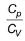value for noble gases is 1.4 iii. Heaviest noble gas present in air is xenon. iv. 6th period starts with caesium and ends with radon.

Solution:value for noble gases is 1.66

QUESTION: 2

### Which statement is false?

Solution:

These three gases were discovered by Travers and Ramsay

QUESTION: 3

### The ionisation potential of hydrogen atom is 13.6 eV. Then ionisation potential of He+ ion is

Solution:

For hydrogen like atom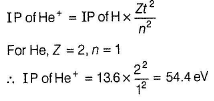QUESTION: 4

Which of the following is/are correct among the following?

i. XeF2 = sp3d, linear
ii. XeF4 = sp3, square planar
iii. XeO4 = sp3, tetrahedral
iv. XeF6 = sp3d2, octahedral

Solution:

Hybridisation of the compounds areQUESTION: 5

The oxidation number of oxygen in O2PtF6 is

Solution:

The structure of O2PtF6 is  O+2 [PtF6]-.
Oxidation tate of O= 2x = +1
x = + 1/2

QUESTION: 6

The structure of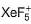ion is

Solution: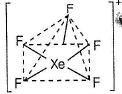QUESTION: 7

The number of (pπ-dπ) π-bonds present in XeO3 and XeO4 respectively are

Solution: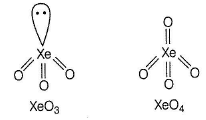QUESTION: 8

XeF6 on reaction with CsF produce

Solution:

The correct answer is option D
CsF + XeF6 → Cs[XeF7]

QUESTION: 9

Which of the following reaction occurs?

Solution:

All the cases are possible reactions.

QUESTION: 10

The wrong statement among the following is

Solution:

He is used in atomic reactor for cooling.

*Multiple options can be correct
QUESTION: 11

Direction (Q. Nos. 11-15) This section contains 5 multiple choice questions. Each question has four choices (a), (b), (c) and (d), out of which ONE or MORE THAN ONE are correct

Q.

Which of the following is/are correct regarding noble gases?

Solution:

*Multiple options can be correct
QUESTION: 12

The correct statements are

Solution:

In dewar’s method, coconut charcoal is used, which adsorb different gases at different temperatures.

*Multiple options can be correct
QUESTION: 13

XeO3 can be prepared by

Solution: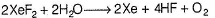*Multiple options can be correct
QUESTION: 14

Pick up the wrong statement(s).

Solution:

lnXeF2, Ip are at equatorial position and XeF4 is square planar while SF4 has see-saw structure

*Multiple options can be correct
QUESTION: 15

The correct statements are

Solution:
QUESTION: 16

Direction (Q. Nos. 16 and 17) This section contains a paragraph, each describing theory, experiments, data, etc. Two questions related to the paragraph have been given. Each question has only one correct answer among the four given options (a), (b), (c) and (d).

Passage

The noble gases have closed-shell electronic configuration and are monoatomic gases under normal conditions. The lower boiling points of the lighter noble gases are due to weak dispersion forces between the atoms and the absence of other interatomic interactions.
Direct reaction of xenon with fluorine leads to the formation of a series of compounds with oxidation numbers +2, + 4, + 6 and + 8. XeF4 reacts violently with water to give XeO3. The compounds of xenon exhibit rich stereochemistry and their geometries can be deduced considering the total number of electron pairs in the valence shell.

Q.

The noble gas compound without lone pair on xenon atom is

Solution: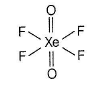QUESTION: 17

The noble gases have closed-shell electronic configuration and are monoatomic gases under normal conditions. The lower boiling points of the lighter noble gases are due to weak dispersion forces between the atoms and the absence of other interatomic interactions.
Direct reaction of xenon with fluorine leads to the formation of a series of compounds with oxidation numbers +2, + 4, + 6 and + 8. XeF4 reacts violently with water to give XeO3. The compounds of xenon exhibit rich stereochemistry and their geometries can be deduced considering the total number of electron pairs in the valence shell.

Q.

Which of the following property decreases from helium to xenon?

Solution:

Rest of properties increases from He to Xe.

QUESTION: 18

Direction (Q. Nos. 18 and 19) This section is based on Statement I and Statement II. Select the correct answer from the codes given below.

Q.

Statement II : α-particles which are equivalent to the atom, reduces the atomic number by 2 and atomic mass by 4 upon decay of mother nucleus.

Solution: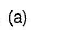QUESTION: 19

Statement I : The structure of XeF4 is square pyramidal.

Statement ll : The hybridisation of XeF4 is sp3d2.

Solution:

Structure of XeF4 is square planar.

QUESTION: 20

Direction (Q. No. 20) Choices for the correct combination of elements from Column I and Column II are given as options (a), (b), (c) and (d) out of which one is correct.

Q.

Match the Column I with Column II and mark the correct option from the codes given below.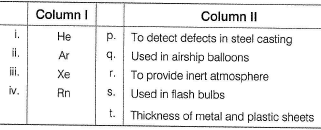Solution: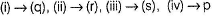*Answer can only contain numeric values
QUESTION: 21

Direction (Q. Nos. 21-25) This section contains 5 questions. When worked out will result in an integer from 0 to 9 (both inclusive).

Q.

Number of lone pairs on the central atom in XeOF4 are

Solution: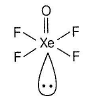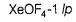*Answer can only contain numeric values
QUESTION: 22

Among XeF2, XeF4, XeF6, XeO3, XeO4, XeO2, XeOF4 the number of compounds that have non-zero dipole moment.

Solution:

Explanation : XeF4 is square planar and XeF2 is linear in shape. The molecules are symmetrical and so the vector sum of the individual dipole moment is zero.

XeO3 is planar in shape. It has 3 dative bonds(coordinate bonds) in one single plane. Taking into consideration that dipole moment is a vector, the 3 dipole moments of 3 bonds being in same plane and of equal magnitude results in a sum of zero. Therefore the dipole moment of net molecule is 0.

*Answer can only contain numeric values
QUESTION: 23

In XeF2, state the total number of electron pairs in its Lewis dot structure.

Solution:

Electron dot structure of XeF2 is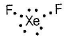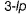*Answer can only contain numeric values
QUESTION: 24

In argon, find the number of electrons with spin value + 1/2.

Solution:

Electronic configuration of Ar is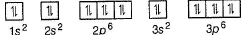Here, 9 electrons each has + 1/2 and - 1/2 value of magnetic moment spin.

*Answer can only contain numeric values
QUESTION: 25

Find the number of unpaired electrons in the fully excited xenon atom.

Solution:

The electronic configuration of Xe is [Kr]4d105s25p6 depending on the energy provided 8 electrons (from 5s and 5p) can go into excited state is 6s, 4f, 5d, 6p, 7s, 5f, 6d, 7p, 8s, etc.